# Barnyard Buddies

A farmer has 8 cows and 10 chickens. The farmer counts all the cow and chicken legs. How many legs are there all together? Show all your mathematical thinking.

Assessment

## Plan

#### Formal Mathematical Language and Symbolic Notation

A student may independently select a printed number line, number chart, ten frames, graph paper, etc. as they work on a task.

## Solutions tabs

### Expert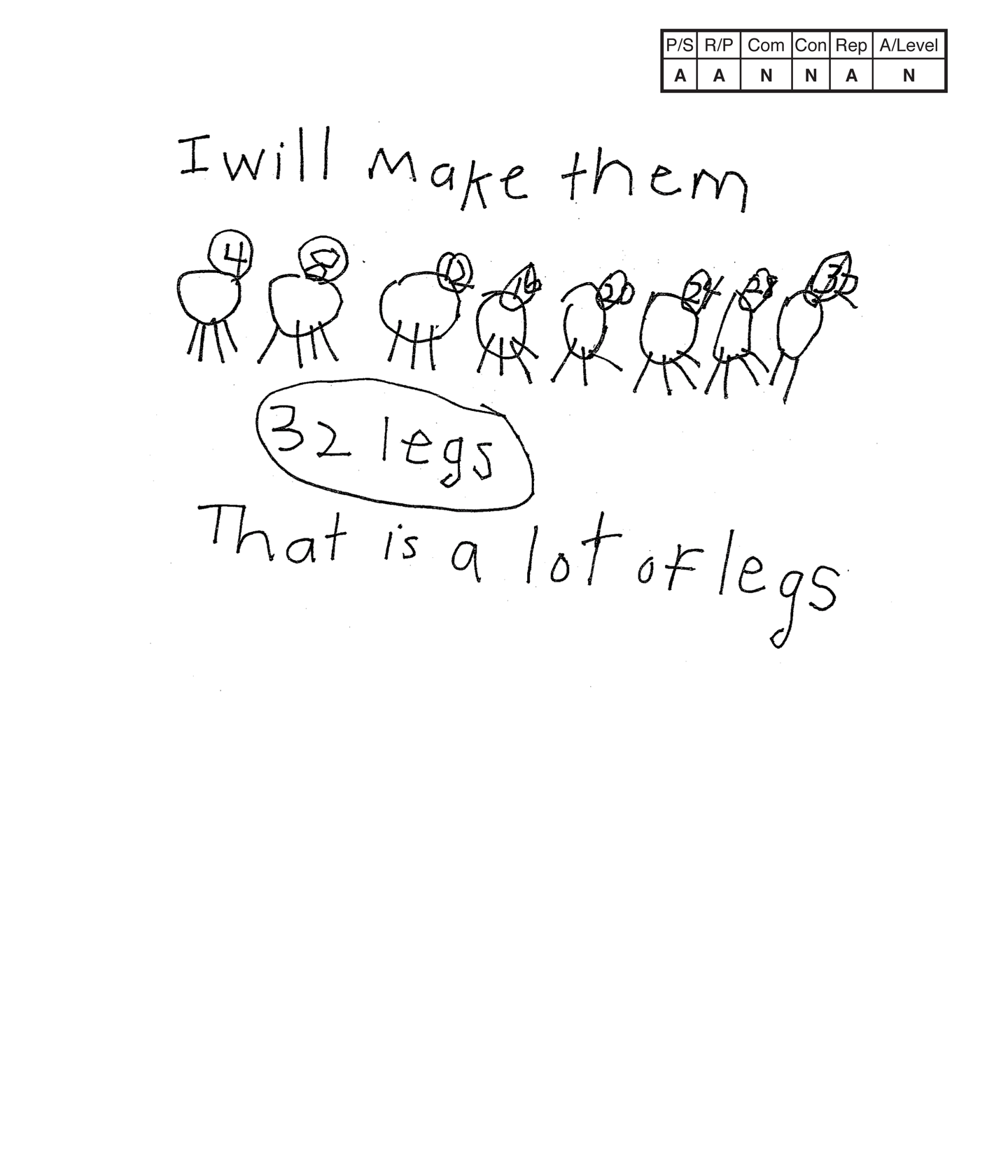This student does not meet the standard.

### Scoring Rationale

#### Apprentice

The student's strategy of using a diagram to show four legs for each cow leads to solving only part of the task. The student's flaw in reasoning is that she/he does not include four legs on each cow and fails to include ten chickens in total legs. The student's answer, "32 legs," is not correct.

#### Apprentice

The student's reasoning has gaps. The student does not define the cows, and the counting of the legs by four does not match the indicated legend—the numbers written in the cow's heads. You have to assume that the last cow is missing two legs and is not a chicken.

#### Novice

The student uses no mathematical language.

#### Novice

The student solves the task and does not make a connection.

#### Apprentice

The student's diagram is appropriate to the task but not accurate. Cows three and five are missing a leg. Cow eight is missing two legs. There is no key, label or scribing to indicate that the student is diagramming cows.

#### Novice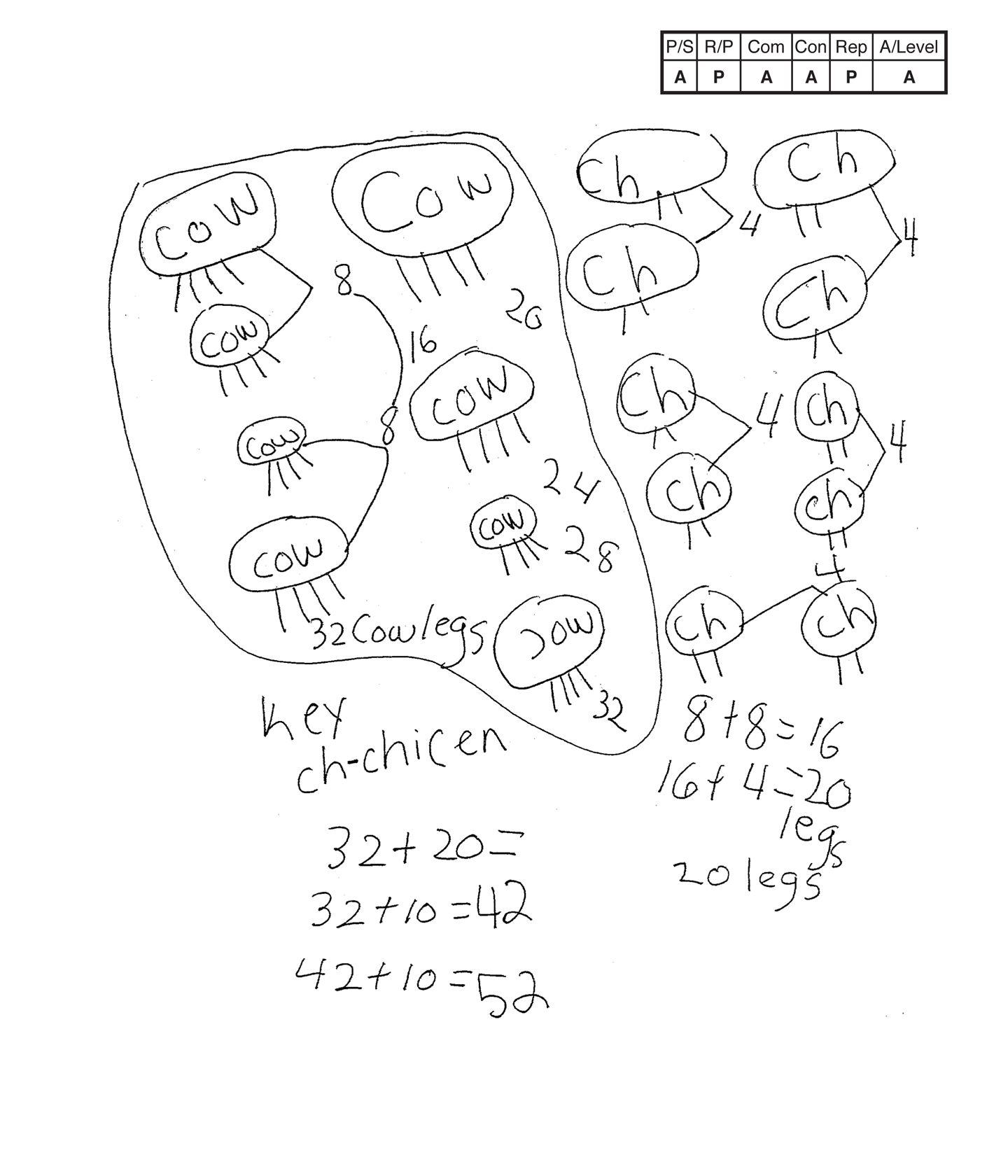,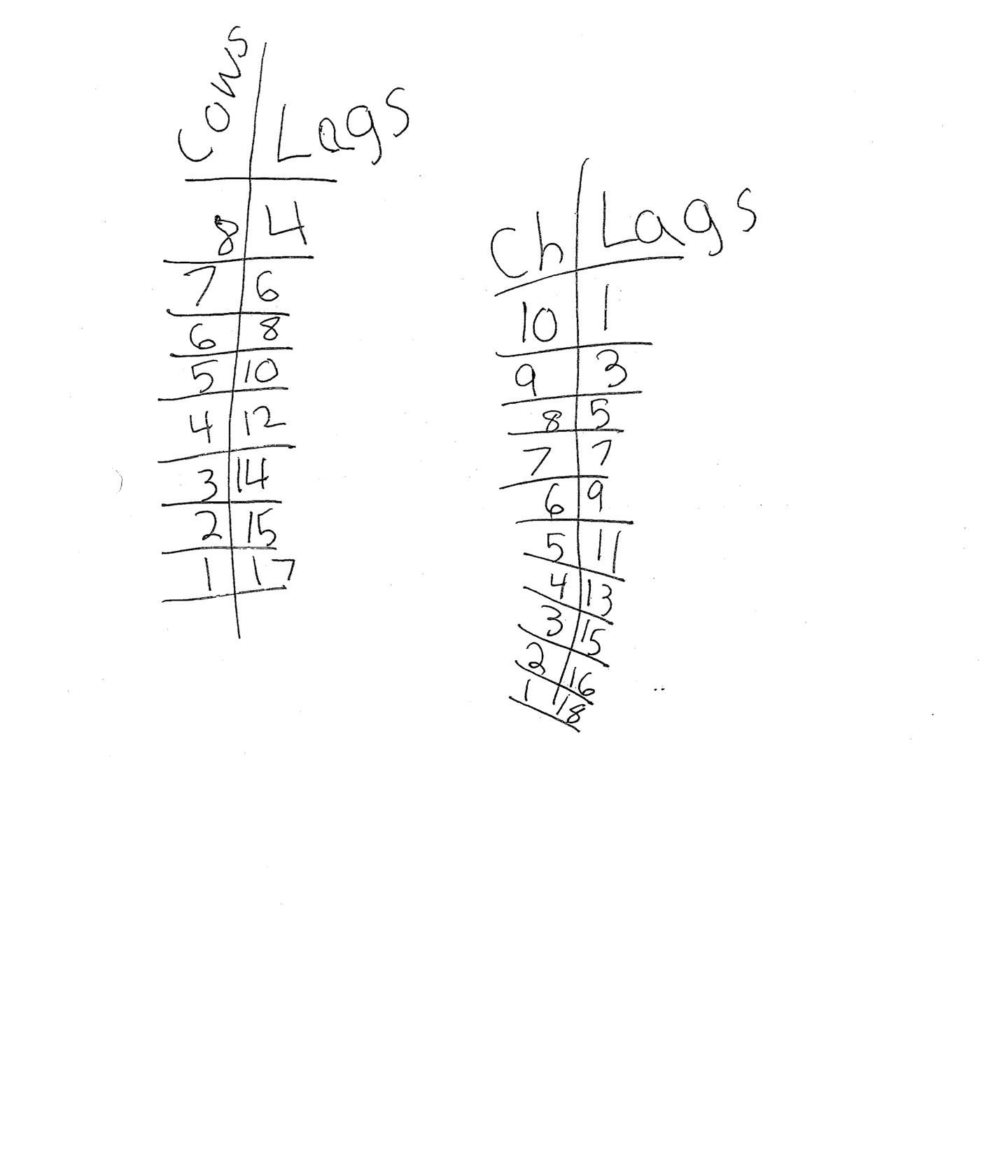,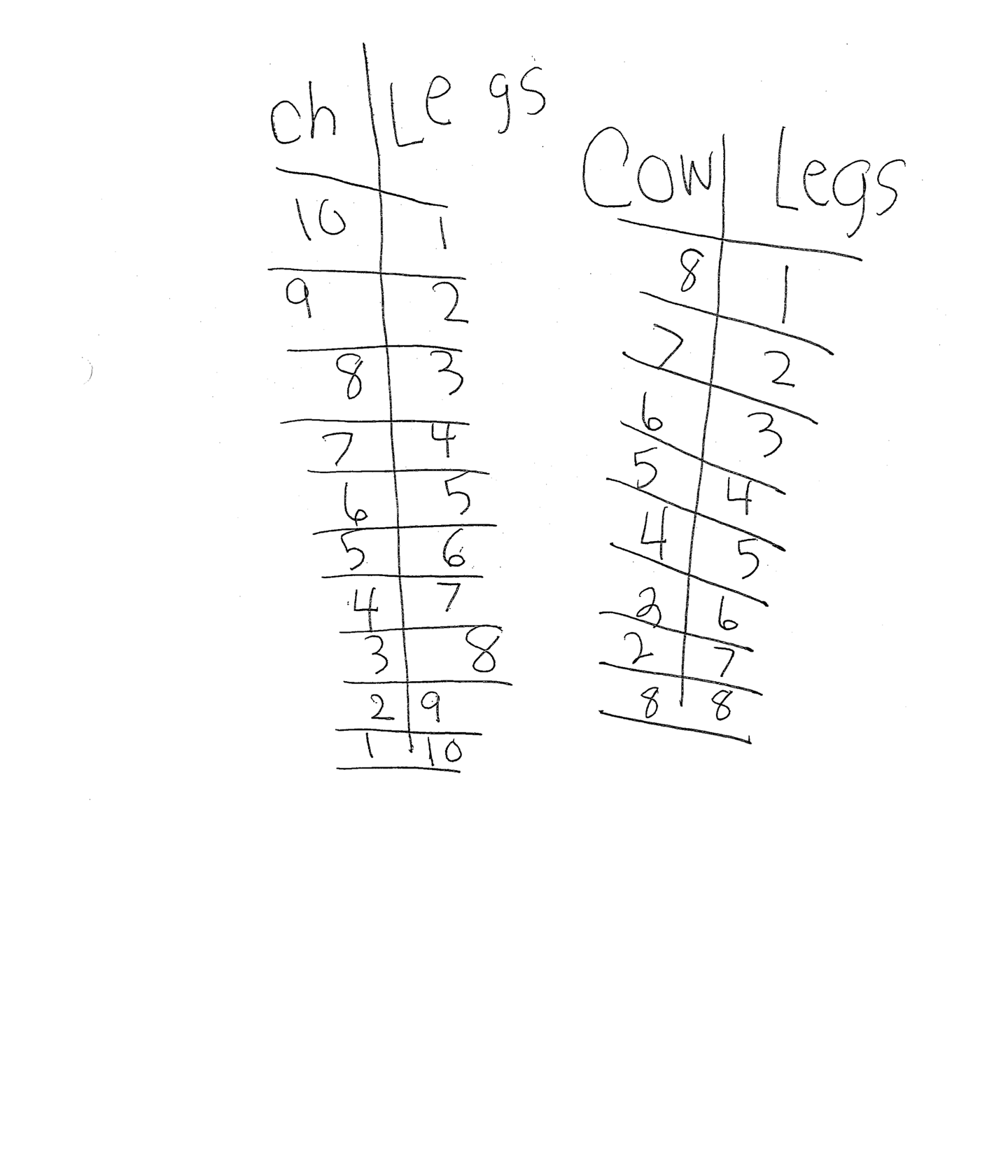This student does not meet the standard.

### Scoring Rationale

#### Apprentice

The student's strategy of using a diagram to show four legs for each cow and two legs for each chicken works to solve this task. The student does not clearly state a correct answer. Most teachers have students highlight, underline, circle, box, etc. the answer.

#### Practitioner

The student's diagrams support their understanding of the underlying mathematical concepts of the task.

#### Apprentice

The student correctly uses the mathematical term key.

#### Apprentice

The student attempts to make a connection. The student attempts four tables but they are incorrect. It is difficult to determine what information the student is trying to represent.

#### Practitioner

The student's diagrams are appropriate to the task and accurate. A key defines "ch" as chickens. Each cow is labeled. The student's tables are not appropriate or accurate to the task. Only one representation has to be appropriate and accurate to earn a Practitioner.

#### Apprentice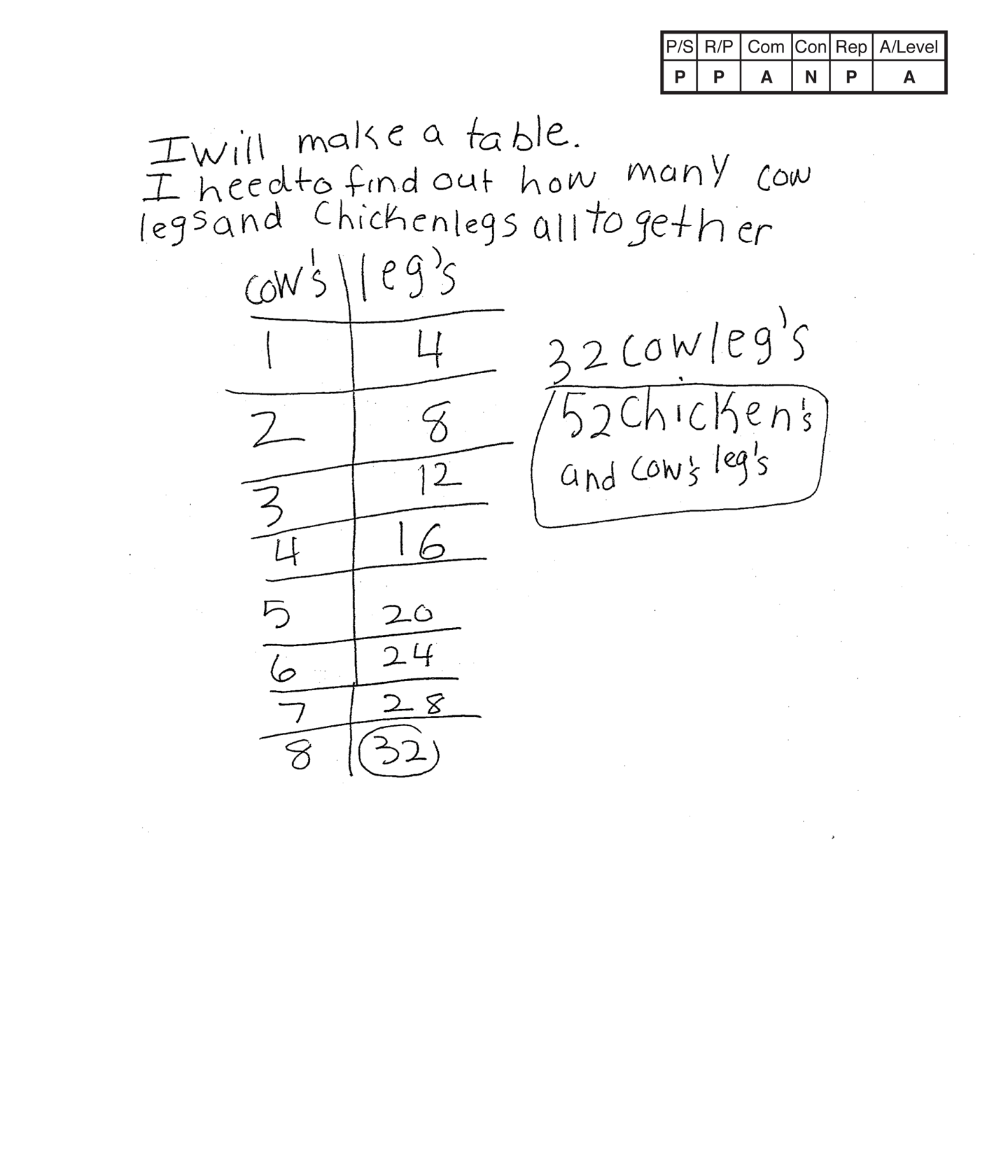,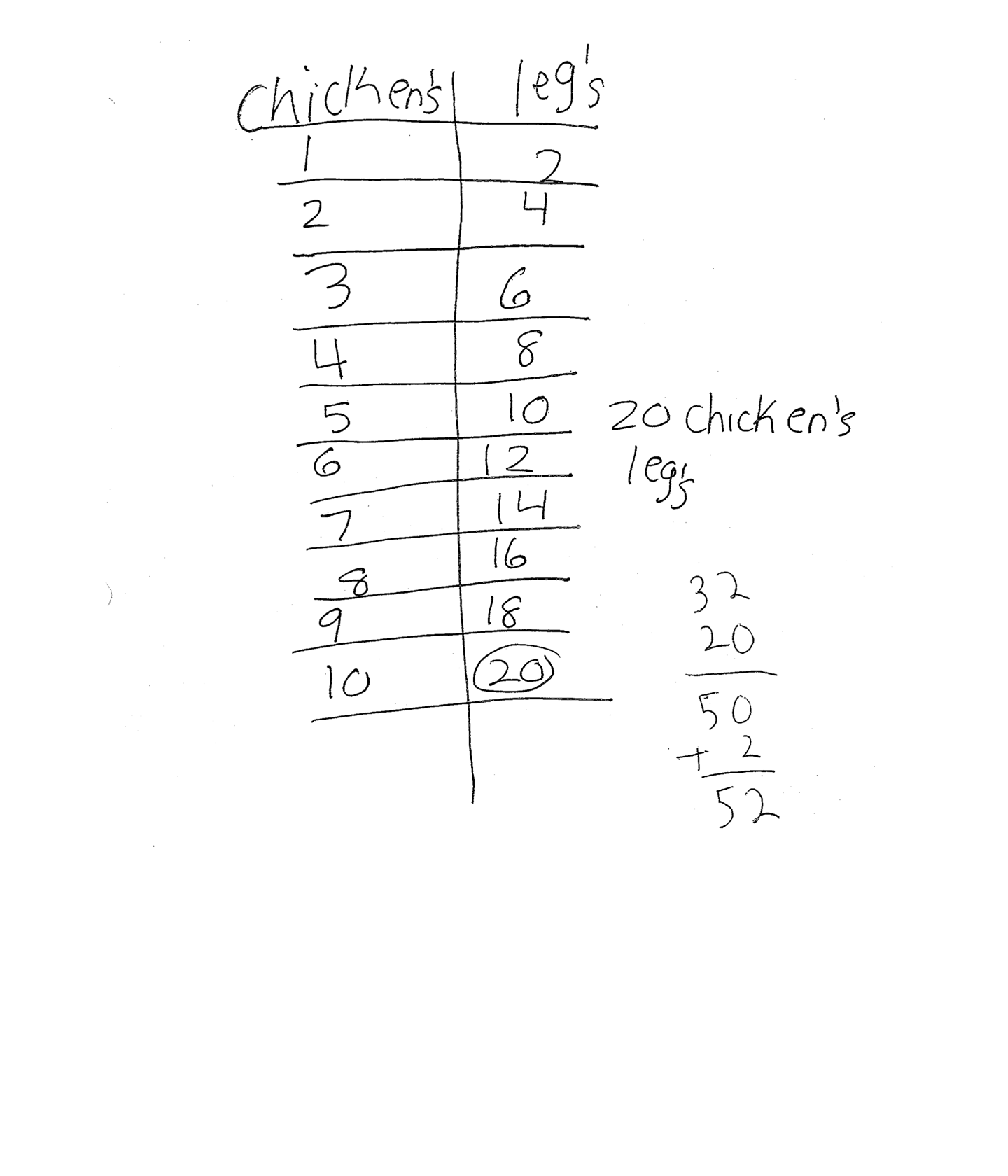This student does not meet the standard.

### Scoring Rationale

#### Practitioner

The student's strategy of making tables of the cow and chicken legs for a total of 52 legs works to solve the task. The student states a correct answer, 52 chicken's and cow's legs.

#### Practitioner

The student's solution clearly shows her/his reasoning and proof. The representations are linked to the text and the presentation is in logical order.

#### Apprentice

The student correctly uses one mathematical term table.

#### Novice

The student does not make any mathematically relevant observation about her/his solution.

#### Practitioner

The student's tables are appropriate to the task and accurate. Both tables have the necessary labels.

#### Apprentice

Note:

The overall achievement level for this piece of student work falls under Exemplars exception to the rule category. If a student has all Apprentice scores or above, but a Novice in "Connections," the student may still receive an achievement level score of Apprentice. To learn more about Exemplars scoring, please refer to the section of your dashboard called "Tools for Success" and click on the link for “Using the Assessment Rubric.”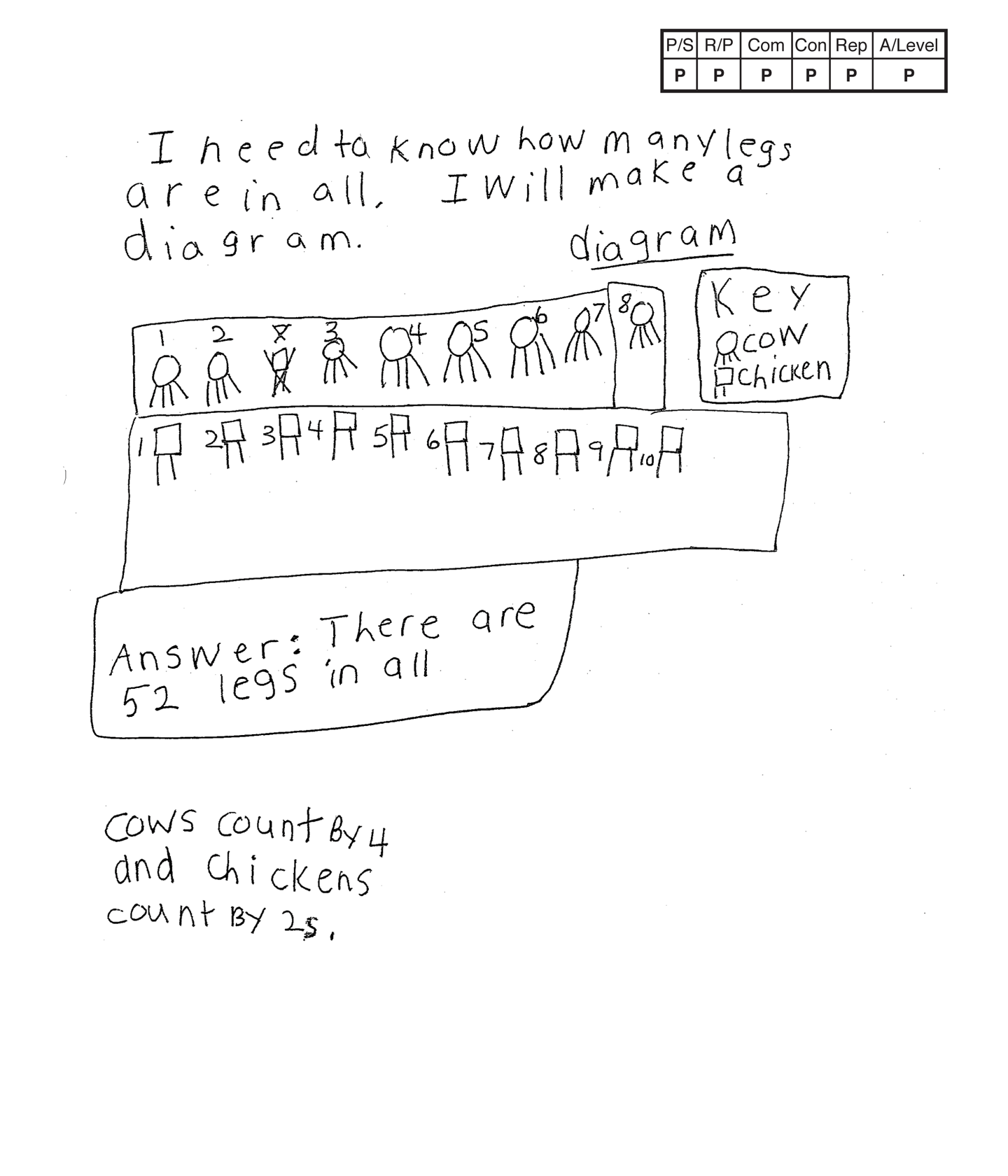This student meets the standard.

### Scoring Rationale

#### Practitioner

The student's strategy of using diagrams to show the eight cows and 10 chickens works to solve the task. The student states a correct answer, "There are 52 legs in all."

#### Practitioner

Arguments are constructed with adequate mathematical basis. The student's correct reasoning is evident in the diagrams and key.

#### Practitioner

The student correctly uses the mathematical terms diagram and key.

#### Practitioner

The student makes the mathematically relevant observations, "cows count by 4 and chickens count by 2s."

#### Practitioner

The student uses appropriate and accurate diagrams to represent the eight cows and 10 chickens. A key is used to define the cows and chickens.

#### Practitioner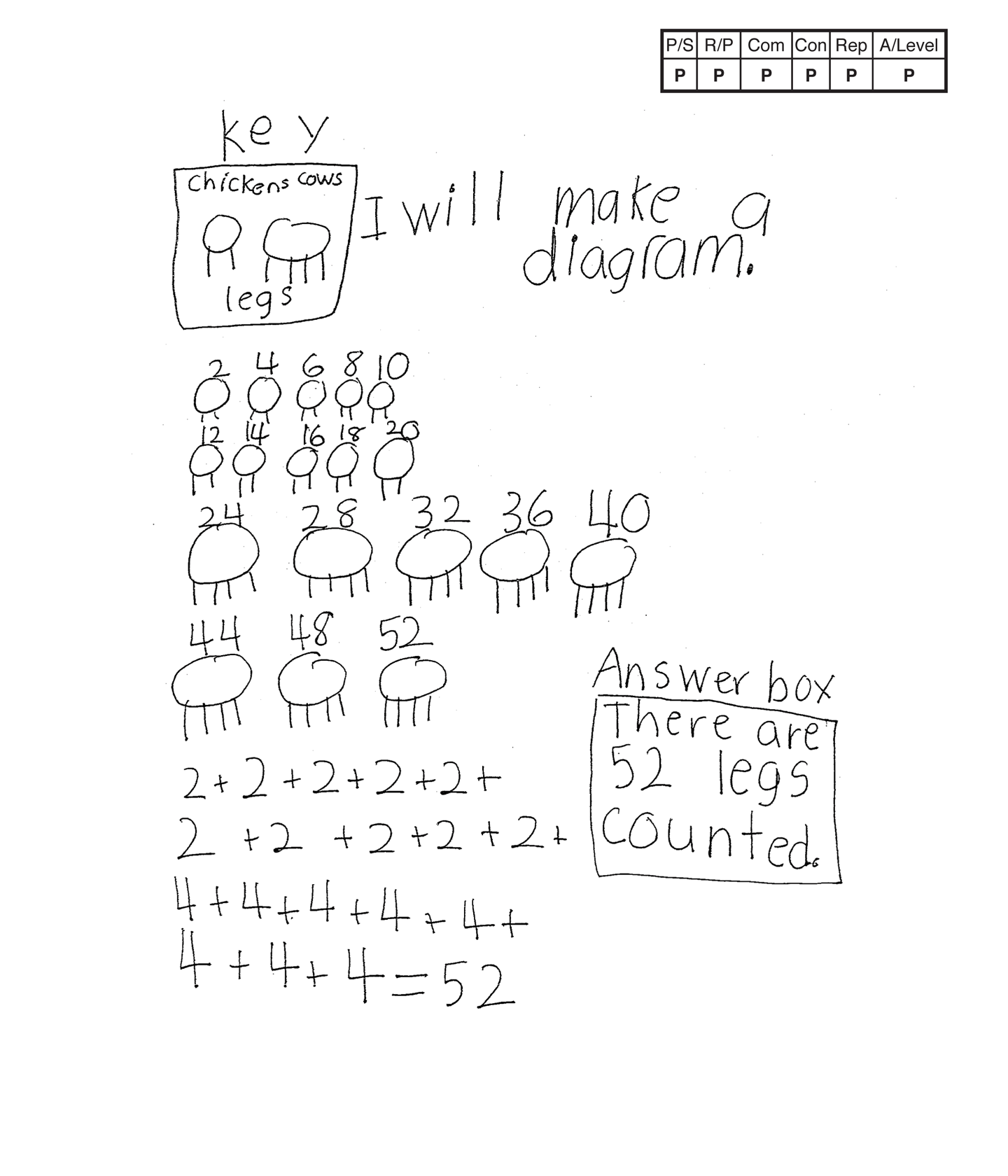This student meets the standard.

### Scoring Rationale

#### Practitioner

The student's strategy of making diagrams to show the number of legs on eight cows and 10 chickens works to solve the task. The student also makes an equation to find a total of 52 legs. The student does not earn Expert for verification because she/he does not compare the two totals of 52 legs.

#### Practitioner

The solution clearly shows the student's reasoning. The representations are linked to the text, results of computation are present, the answer is indicated, and the presentation is in logical order.

#### Practitioner

The student correctly uses the mathematical terms key and diagram.

#### Practitioner

The student solves the task and then uses a different process to solve the same task. The student uses a number sentence to find a total of 52 legs.

#### Practitioner

The student's diagrams are appropriate to the task and accurate. A key defines the cow and chicken legs.

#### Practitioner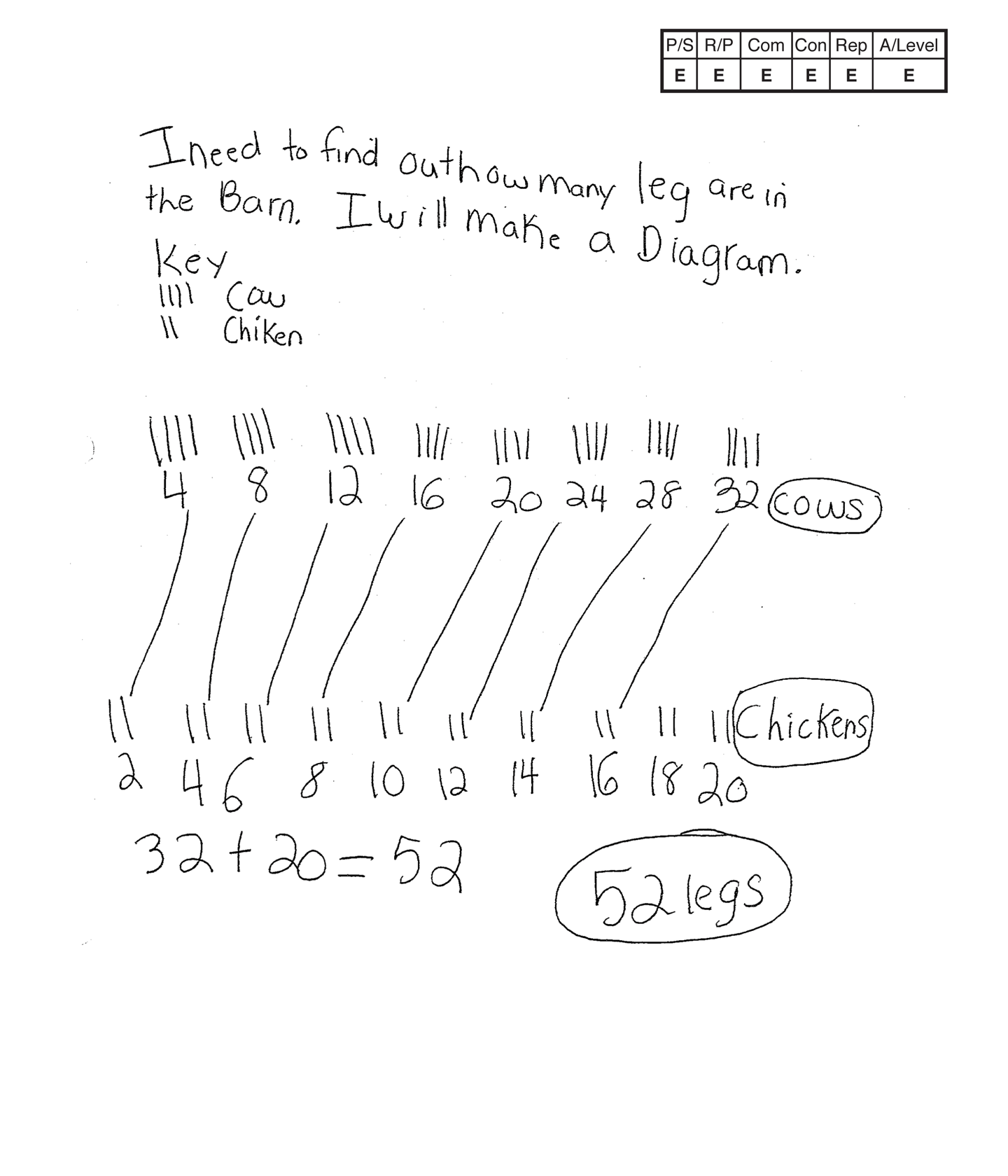,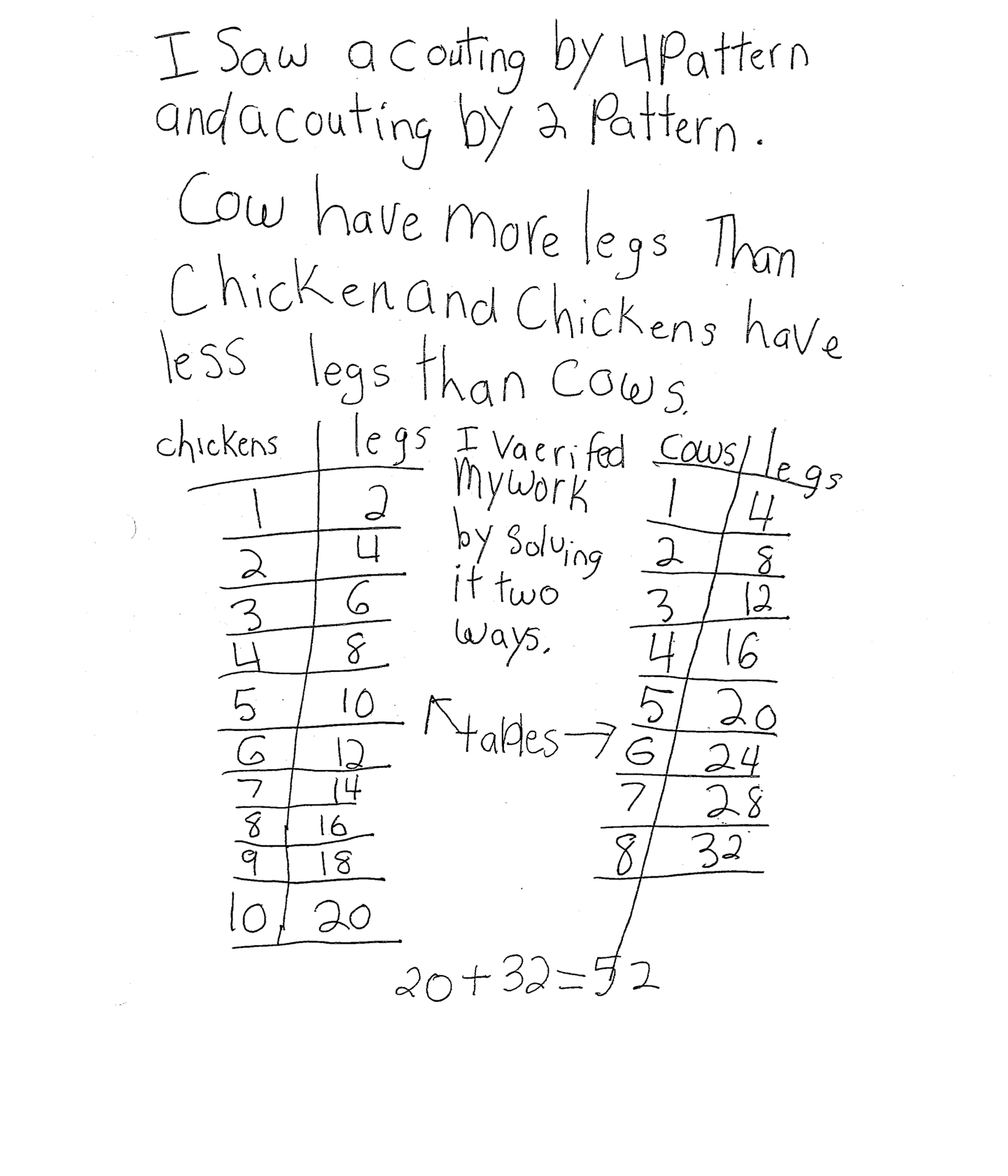This student exceeds the standard.

### Scoring Rationale

#### Expert

The student's strategy of making a diagram to show four legs on each cow and two legs on each chicken and finding a total of 52 legs works to solve the task The student then verifies her/his solution by making tables to find a total of 52 legs and stating, "I verified my work by solving it two ways."

#### Expert

The student's solution justifies and supports the decisions made and the conclusions reached.

#### Expert

The student correctly uses the mathematical terms key, diagram, pattern, more than, less than, table.

#### Expert

The student solves the task and then uses a different process to verify her/his solution. The student uses a table to show the chicken legs and a table for the cow legs and states, "I verified my work by solving it two ways." (Expert Connection) The student makes a number of Practitioner Connections as well: The student makes the relevant observations, "Cow have more legs than chicken and chickens have less legs than cows." The student also identifies patterns. "I saw a counting by 4 Pattern and a counting by 2 Pattern."

#### Expert

The student's diagrams and tables are appropriate to the task and accurate. A key and all necessary labels are provided. The student's representations are constructed to clarify/verify the solution.

#### Expert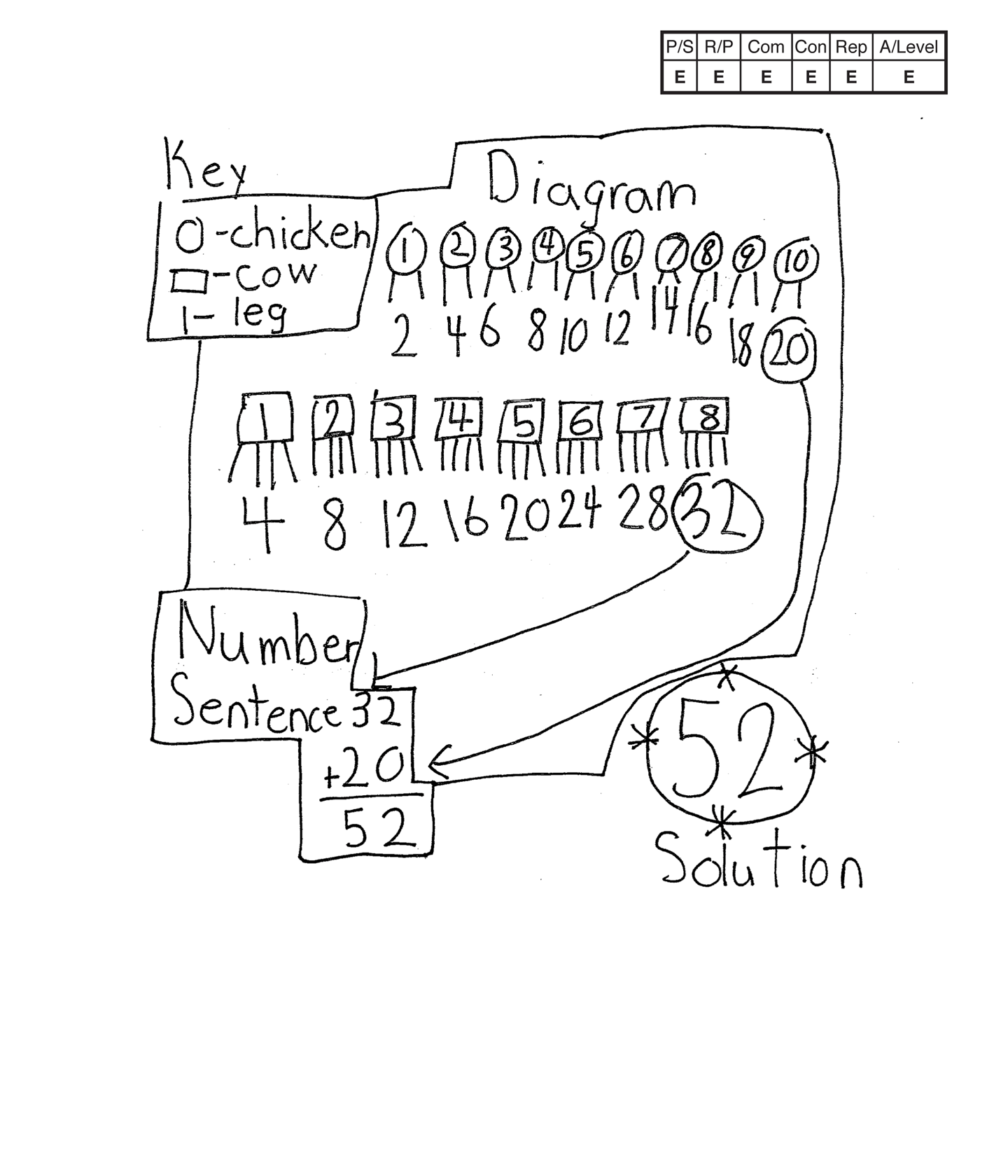,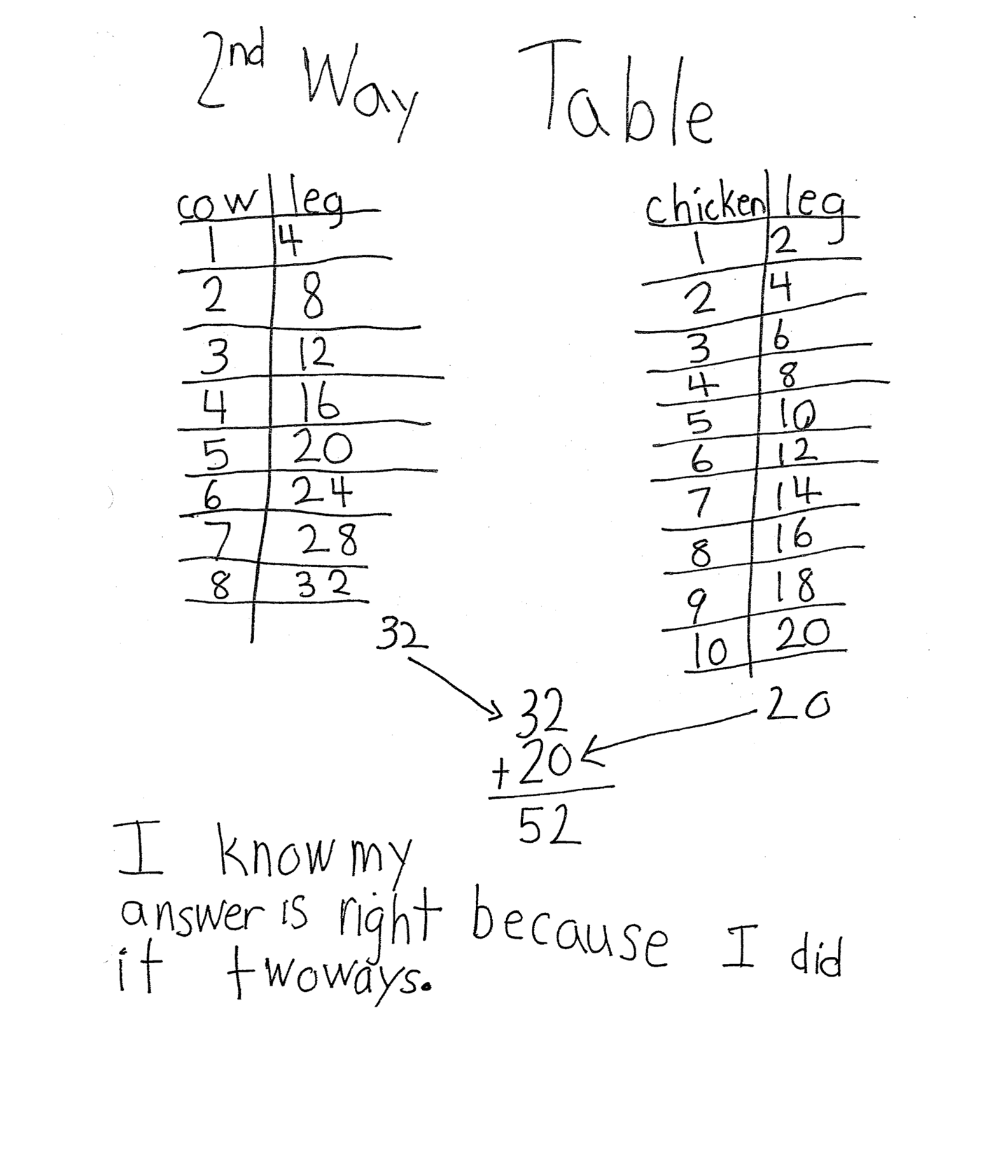This student exceeds the standard.

### Scoring Rationale

#### Expert

The student's strategy of making a diagram to show four legs on each cow and two legs on each chicken and finding a total of 52 legs works to solve the task. The student uses an alternate strategy to verify her/his solution.

#### Expert

The student's solution shows how the task was solved and the reasoning used. The representations are linked to the text, results of computation are present, and the presentation is in logical order.

#### Expert

The student correctly uses the mathematical terms key, diagram, 2nd, table.

#### Expert

The student solves the problem with a diagram and then uses a different process to solve the same task. The student uses a table to verify her/his solution and states, "I know my answer is right because I did it two ways."

#### Expert

The student's diagrams and tables are appropriate and accurate. A key and all necessary labels are provided. The student uses both representations to clarify her/his answer.